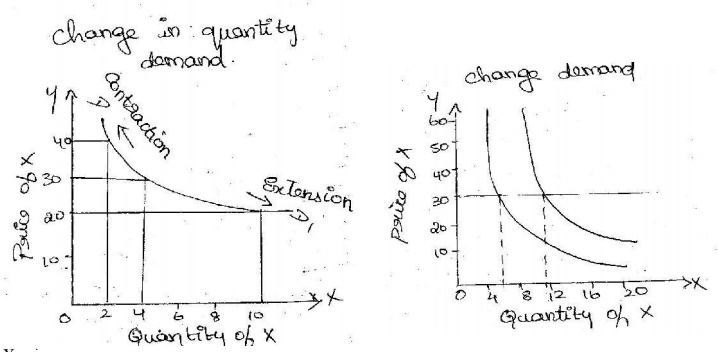Home | Difference Between Changes in Demand and Changes In Quantity Demanded

# Difference Between Changes in Demand and Changes In Quantity DemandedChange in Quantity demanded: A demand curve is the graphic representation of the law of demand. Movement alo ng a demand curve is caused b y a change in the own price of the commodity.

DIFFERENCE BETWEEN CHANGES IN DEMAND AND CHANGES IN QUANTITY DEMANDED

Change in Quantity demanded: A demand curve is the graphic representation of the law of demand. Movement alo ng a demand curve is caused b y a change in the own price of the commodity. Such as change is called extension and contraction of demand. This means movement on the demand curve resulting in extension of demand. Demand contracts as price of good increases. This movement on a demand curve is known as change in quantity demanded. This is be explained with the help of a diagramX axis -------Quantity of X

Y axis---------price of X

DD ------------demand curve

Explanation:

The figure shows that as the price increases the demand decreases & as the price decreases the demand for the commodity increases.

Change in demand: A shift of the demand curve is brought about b y change in factors other thanthe?own'priceeg.Itchangesinfactorlikeincomesoftheconsumerpricesofsubstitute products, percentage of women going out to work etc, this is known as change in demand.

This is explained with the help of a diagram.X axis -------Quantity of X

Y axis---------price of X

DD ------------demand curve

DD1 ------------Shift in Demand curve

Explanation:

The purchasing power of the consumer increases at each given price he starts buying more of the co mmodity. This tendency of the consumer leads to shifts in this demand curve. S imilar results will emerge if other determinants like price of related goods, tastes, etc, change. All the??other determinants?aretherefore, called shift factors, which lead to change in demand.

Study Material, Lecturing Notes, Assignment, Reference, Wiki description explanation, brief detail
Civil : Engineering Economics and Cost analysis : Demand and Schedule : Difference Between Changes in Demand and Changes In Quantity Demanded |